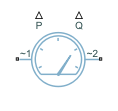# Power Sensor (Three-Phase)

Three-phase ideal active and reactive power sensor

• Library:
• Simscape / Electrical / Sensors & Transducers

•## Description

The Power Sensor (Three-Phase) block implements an ideal sensor for active and reactive power measurement in balanced or unbalanced three-phase branches.

For balanced branches, the block returns the correct value of the active and reactive power at all time instants. This is the preferred option for a load-flow analysis.

For unbalanced branches, to estimate the magnitude and phase, the block measures the phasors of the three voltages and currents independently by using a one-moving-period Fourier transform. For this reason, the output magnitude and phase are zero during the first period.

### Unbalanced Branches Equations

The equivalent balanced phase voltage, current, and apparent power are:

`$\begin{array}{l}{V}_{e}=\frac{1}{3}\sqrt{{V}_{ab}{}_{{}_{RMS}}^{2}+{V}_{bc}{}_{{}_{RMS}}^{2}+{V}_{ca}{}_{{}_{RMS}}^{2}}\\ {I}_{e}=\sqrt{\frac{{I}_{a}{}_{{}_{RMS}}^{2}+{I}_{b}{}_{{}_{RMS}}^{2}+{I}_{c}{}_{{}_{RMS}}^{2}+\rho {I}^{2}{}_{{n}_{RMS}}}{3}}\\ {S}_{e}=3{V}_{e}{I}_{e}\end{array}$`

where

• VabRMS, VbcRMS, and VcaRMS are the three phase-to-phase RMS voltages.

• IaRMS, IbRMS, and IcRMS are the RMS currents that flow through each of the three branches.

• ρ is the ratio of neutral conductor resistance over phase conductor resistance, specified by the Neutral conductor resistance divided by phase conductor resistance parameter.

• InRMS is the RMS neutral current.

The active power is then defined by:

`$P={V}_{{a}_{RMS}}{I}_{{a}_{RMS}}\mathrm{cos}\left({\phi }_{{V}_{a}}-{\phi }_{{I}_{a}}\right)+{V}_{{b}_{RMS}}{I}_{{b}_{RMS}}\mathrm{cos}\left({\phi }_{{V}_{b}}-{\phi }_{{I}_{b}}\right)+{V}_{{c}_{RMS}}{I}_{{c}_{RMS}}\mathrm{cos}\left({\phi }_{{V}_{c}}-{\phi }_{{I}_{c}}\right),$`

where:

• VaRMS, VbRMS, and VcRMS are the voltages of phase a, b, and c respectively.

• φVa, φVb, and φVc are the phase shifts of the a-phase, b-phase, and c-phase voltages.

• φIa, φIb, and φIc are the phase shifts of the a-phase, b-phase, and c-phase currents.

To compute the reactive power, the block first calculates its absolute value and then finds the sign by looking at the sign of the phase shift:

`$\begin{array}{l}abs\left(Q\right)=\sqrt{{S}_{e}^{2}-{P}^{2}}\\ sign\left(Q\right)=sign\left(\phi \right)\\ Q=abs\left(Q\right)*sign\left(Q\right)\end{array}$`

where φ = φV* - φI*. The * symbol denotes the central phase in the [-π,π] range.

If the block is in a network that is compatible with the frequency-time simulation mode, you can perform a load-flow analysis on the network. A load-flow analysis provides steady-state values that you can use to initialize a machine.

For more information, see Perform a Load-Flow Analysis Using Simscape Electrical and Frequency and Time Simulation Mode.

## Ports

expand all

### Output

expand all

Physical signal port associated with the active power measurements.

Physical signal port associated with the reactive power measurements.

## Parameters

expand all

Whether to model composite or expanded three-phase ports.

Composite three-phase ports represent three individual electrical conserving ports with a single block port. You can use composite three-phase ports to build models that correspond to single-line diagrams of three-phase electrical systems.

Expanded three-phase ports represent the individual phases of a three-phase system using three separate electrical conserving ports.

Type of load. Select `Balanced` if the three-phase branches are balanced, `Unbalanced` if the branches are not balanced.

Rated frequency.

#### Dependencies

To enable this parameter, set Load Type to `Unbalanced`.

Whether the downstream configuration is with or without neutral.

#### Dependencies

To enable this parameter, set Load Type to `Unbalanced`.

Ratio of neutral conductor resistance over phase conductor resistance, $\frac{{R}_{neutral}}{{R}_{phase}}.$.

#### Dependencies

To enable this parameter, set Downstream configuration to ```With neutral```.

 Willems, Jacques, "The IEEE standard 1459: What and why?", 2010 IEEE International Conference on Applied Measurements for Power Systems, Proceedings: 41-46.